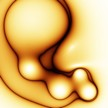# AI-Portal Artificial Intelligence for Enthusiasts

## Genetically evolved images Part 1

We can define an image representation using parametrized functions in the following way:

f(x,y) is the color of the pixel (x,y), given that very small numbers are darker colors, and very high numbers are bright colors. To obtain different colors, we can start from predefined set of 3 basic colors, and multiply them by the values give by function f. Then we can tweak the output by playing with hue and saturation. We use a clamping operator to restrict the values to [0,255] range.

This is an enhancement to the mathematical 2D representation of a function that we use in elementary school.

A few examples can contain: a constant function will represent into a uniform screen of a single color. A sinus function would then create some stripes:We can then get into more complicated images, like the following:The question at hand is how to get more images, and how to create such functions?

We will use the genetic algorithms, mainly for artificial evolution. The phenotype is represented by the resulting image itself, and the genotype is the mathematical function representation. If we are given different genotypes, we can cross-over and mutate them using genetic algorithms, in few different epochs, to obtain more complicated images. We can start from a sinus , identity, power, sum, subtraction functions, then try logarithm, etc.
This type of image generation is called evolutionary method using artificial intelligence.
All images are taken from Blot website.
Get more Joomla!® Templates and Joomla!® Forms From Crosstec